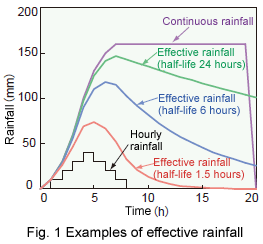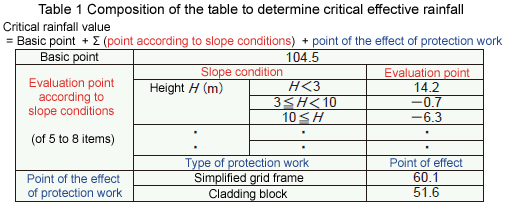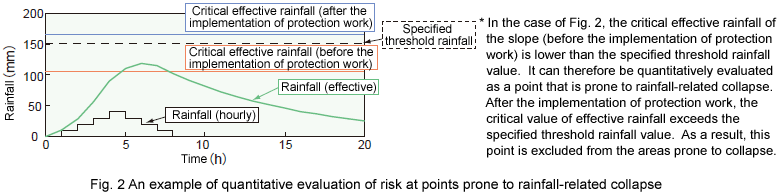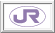1. Creation of Evaluation Table for Risk of Slope Collapse based on Effective Rainfall
 Creation of evaluation table for risk of slope collapse based on effective rainfall. Quantitative evaluation of slope prone to collapse by rainfall in using the table.Train operation is now controlled in rainy conditions on a rainfall index that combines continuous rainfall and hourly rainfall. However, the introduction of effective rainfall as the new rainfall index is now under consideration. Effective rainfall is an index representing the volume of water that stays in the ground, which is determined for different half-life values (Fig. 1). The half-life is the length of time that represents the effects of rain in the past (i.e. the longer the half-life is, the larger the volume of water is accumulated in the ground).

If a slope along a railway line collapses before rainfall reaches the specified threshold value for train operation control (the limit rainfall), train derailment, overturning or other serious damage may occur. It is therefore essential to estimate the risk of slope collapse in using the rainfall index for train operation control, and to find slopes prone to collapse in comparing the estimated risk with the limit rainfall.

An evaluation table is thus created which allows to estimate the risk of slope collapse by the effective rainfall. It determines the half-live (1.5, 6 or 24 hours), taking into account slope types (embankment or cutting) (Table 1). The table makes it possible to calculate the critical effective rainfall (the value beyond which the risk of slope collapse sharply increases) according to the conditions of the object slope and to evaluate quantitatively slope prone to collapse, comparing this value with those of other slopes or with the limit rainfall (Fig. 2). The developed criteria for evaluation will be readily applicable when the effective rainfall is introduced for the train operation control in raining. An evaluation table can thus be established in case a different rainfall index is introduced.R&D > Major Results of Research and Development in Fiscal 2006 > I Safety/ReliabilityRTRI HOME